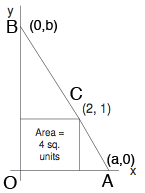SEARCH HOMEMath Central Quandaries & QueriesQuestion from Charlene, a teacher: What is the slope of this line?Hi Charlene,

I reproduced the diagram you sent and added some labels. You are asked to find the slope of the line passing through $A$ and $B.$I was confused at first with the statement "Area = 4 sq. units" but I think it means that the area of the triangle $ABO$ is 4 square units. If this is true then what do you know about $a \times b?$ Use the points $A$ and $B$ to write an expression for the slope of the line through $A$ and $B.$ Use the points $A$ and $C$ to write an expression for the slope of the line through $A$ and $C.$ Use the points $B$ and $C$ to write an expression for the slope of the line through $B$ and $C.$ These three slopes are all the same so use the expressions you just developed and what you know about $a \times b$ to solve for $a$ and $b.$

PennyMath Central is supported by the University of Regina and The Pacific Institute for the Mathematical Sciences.